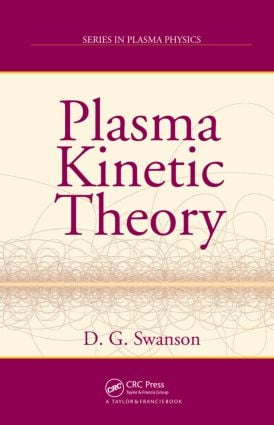Plasma Kinetic Theory

1st Edition

Chapman and Hall/CRC

344 pages | 39 B/W Illus.

Hardback: 9781420075809
pub: 2008-05-13
\$145.00
x
eBook (VitalSource) : 9780429149153
pub: 2008-05-13
from \$72.50

FREE Standard Shipping!

Description

Developed from the lectures of a leading expert in plasma wave research, Plasma Kinetic Theory provides the essential material for an introductory course on plasma physics as well as the basis for a more advanced course on kinetic theory. Exploring various wave phenomena in plasmas, it offers wide-ranging coverage of the field.

After introducing basic kinetic equations and the Lenard–Balescu equation, the book covers the important Vlasov–Maxwell equations. The solutions of these equations in linear and quasilinear approximations comprise the majority of kinetic theory. Another main topic in kinetic theory is to assess the effects of collisions or correlations in waves. The author discusses the effects of collisions in magnetized plasma and calculates the different transport coefficients, such as pressure tensor, viscosity, and thermal diffusion, that depend on collisions.

With worked examples and problem sets that enable sound comprehension, this text presents a detailed, mathematical approach to applying plasma kinetic theory to diffusion processes in plasmas.

INTRODUCTION

Overview

Plasma frequency

Debye length

Plasma parameter

Distribution functions

Cyclotron frequencies

Collisions

Particle drifts

Waves

BASIC KINETIC EQUATIONS

The Klimontovich equation

The Liouville equation

System ensembles

THE LENARD–BALESCU EQUATION

Bogolyubov’s hypothesis

Solution via Fourier and Laplace transforms

The Fokker–Planck equation

Dynamic friction and diffusion

THE VLASOV-MAXWELL EQUATIONS

Electrostatic waves in an unmagnetized plasma

Effects of collisions on Landau damping

The Debye potential

WAVES IN A MAGNETIZED HOT PLASMA

The hot plasma dielectric tensor

Electrostatic waves

Velocity space instabilities

Conservation of energy and power flow

Collisional effects

Relativistic plasma effects

MOMENT EQUATIONS AND FLUID PLASMAS

Moments of the distribution function

The fluid equations

Low frequency waves

High frequency waves

TRANSPORT IN A NONUNIFORM GAS

Boltzmann equation

Collision symmetries

Collision theorems

The equilibrium state

The mean free time theory

The formal theory of kinetic processes

Results of the variational procedure

TRANSPORT IN A NONUNIFORM BINARY GAS

The Boltzmann equations

The equations of hydrodynamics

The collision terms

The equilibrium state

The formal theory of kinetic processes

The variation method

Results

TRANSPORT WITH A FINITE MAGNETIC FIELD

Boltzmann equations

The magnetohydrodynamic (MHD) equations

The formal theory of kinetic processes

Solutions for the electrical conductivity

Thermal conductivity and diffusion

The pressure tensor

Summary of results

APPENDIX A: MATHEMATICAL FUNCTIONS

APPENDIX B: COLLISION INTEGRALS

APPENDIX C: NOTATION AND LIST OF SYMBOLS

Bibliography

Index